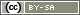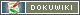# Randomization per Block

Usually, all subjects are randomly distributed among all experimental conditions (experimental groups) (Single Factorial Design). Random assignment to experimental conditions provides the best chance that experimental groups will be equal in terms of known and unknown predispositions – within the bounds of statistical selection error.

However, the statistical selection error may mean that the experimental group is not completely independent of subject characteristics. For example it is possible that there are more women in the control group than in the experimental group. This is problematic when a person characteristic serves as a quasi-experimental factor in the experiment.

In this case it can be helpful to generate a randomization per block. This means that subjects are first divided into groups (blocks) based on a characteristic and that assignment to experimental conditions is then made within these blocks.

Note: Block randomization is only relevant if “draw without reserve” has been used to ensure that all experimental conditions are drawn equally often. In SoSci Survey, the “equal distribution” is the default for a Random Generator.

## Implementation in SoSci Survey

In SoSci Survey you use a separate random number generator for each block. This ensures that the draws in one block are independent of the draws in the other blocks.

The procedure is as follows:

1. Ensure that the random number generator makes a “uniformly distributed” draw – either at the time of the draw (default) or a uniform distribution in completed questionnaires.
2. Create copies of the random generator so that you have as many random generators as blocks (Manage Questions and use as Templates).
3. Create a PHP-Filter, which embeds the appropriate random generator depending on the person characteristics (block membership) (below: include the random generator).
4. If you display the stimulus via PHP code (Randomization with PHP-Code),

## Include the Random Generator

If you have followed the instructions for Randomization, you have always dragged and dropped the randomizer onto a questionnaire page. You do not need this step by block randomisation because here the random generator has to be integrated via `show()` or `question()`.

Important: Do not place any of your random generators in the questionnaire. If you have already dragged a random generator into the questionnaire, then please remove it again from the corresponding questionnaire page under Create questionnaire.

The PHP code to include the proper randomizer follows the same basics as the IF filters presented in the Filter Questions (PHP Filters) chapter.

In the following example, the gender of a person was asked on page 2 of the questionnaire (variable “SD02”) and you want to randomize in two blocks: Men (Code 2) and the others (Answercodes 1 and 3). Therefore two random generators RG01 for men and RG02 for all others were created. The following PHP code is placed where the assignment to the trial condition is to be made, but no earlier than page 3.

```if (value('SD01') == 2) {
// Include random generator RG01 (men)
show('RG01');
} else {
// Include random generator RG02 (for all others)
show('RG01');
show('RG02');
}```

Note: If the HTML code for displaying the stimulus is already stored in the random generators (single factorial design), you do not need any further PHP code and you are finished with that. If, on the other hand, you are displaying the stimulus using PHP code, please continue following these instructions.

Note: In principle, you can also divide the respondents into several blocks, e.g., by age and education, if these characteristics are relevant for the design. Please be aware, however, that more than 6 blocks are only useful for large numbers of cases. Estimate in advance the number of cases per block.

## Display the Stimulus per PHP-Code

If you do not display the stimulus via HTML code in the randomizer but via PHP code, then you have used the variable of the randomizer for this up to now (Randomization with PHP-Code), e.g.

```if (value('RG01') == 2) {
// display text-stimulus A
text('ST01');
} elseif (value('RG01') == 3) {
// display text-stimulus B
text('ST02');
}```

Now different random generators are used depending on the block. Therefore, create an internal variable in your question catalog.

Note: The question type “internal variables” provides for several variables per question. Therefore a single internal variable carries a longer identifier (e.g. “RG04_01”) than the variable of a random generator (“RG01”).

The following example assumes that the question of the type “internal variables” has the identifier “RG04” and has one item (“RG04_01”). The above PHP code to include the correct random number generator is now supplemented with PHP code that copies the drawn random number into this new variable using `put()`.

```if (value('SD01') == 2) {
// Include random generator RG01 (men)
show('RG01');
put('RG04_01', value('RG01'));
} else {
// Include random generator RG02 (all others)
show('RG02');
put('RG04_01', value('RG02'));
}```

Regardless of which random generator was used, the drawn code is now (also) stored in the variable RG04_01. This variable can then be used to present the appropriate stimulus.

```if (value('RG04_01') == 2) {
// display text-stimulus A
text('ST01');
} elseif (value('RG04_01') == 3) {
// display text-stimulus B
text('ST02');
}```
en/create/randomization-per-block.txt · Last modified: 03.08.2021 10:51 by sophia.schauer